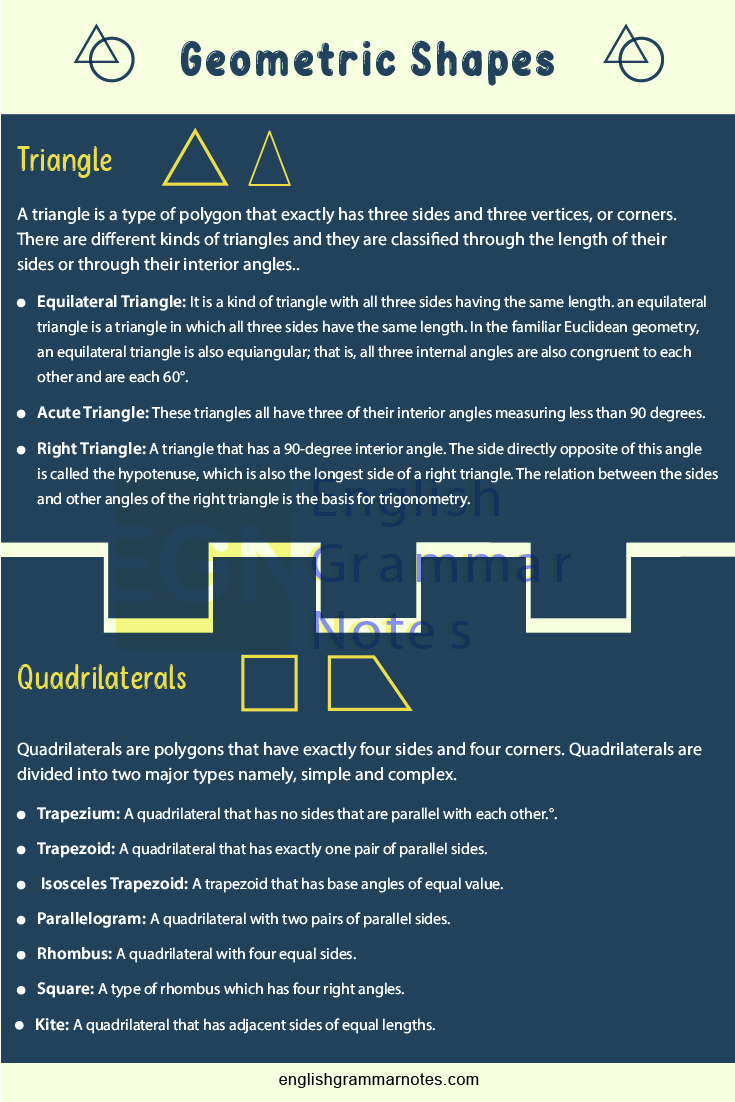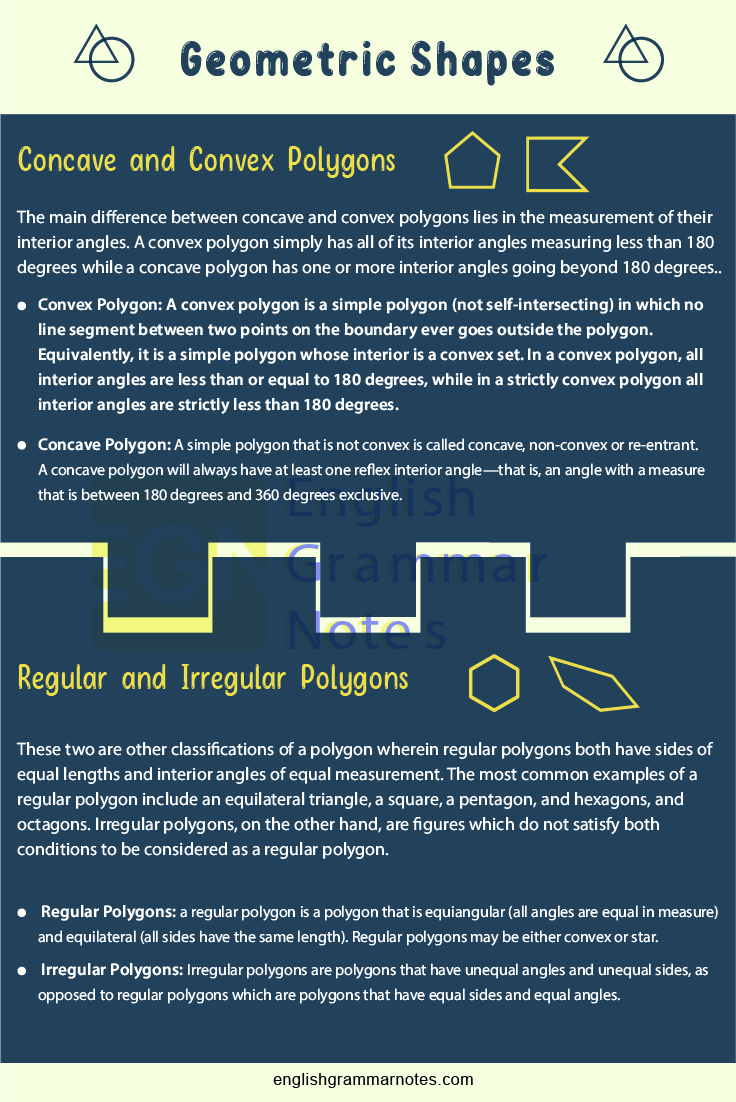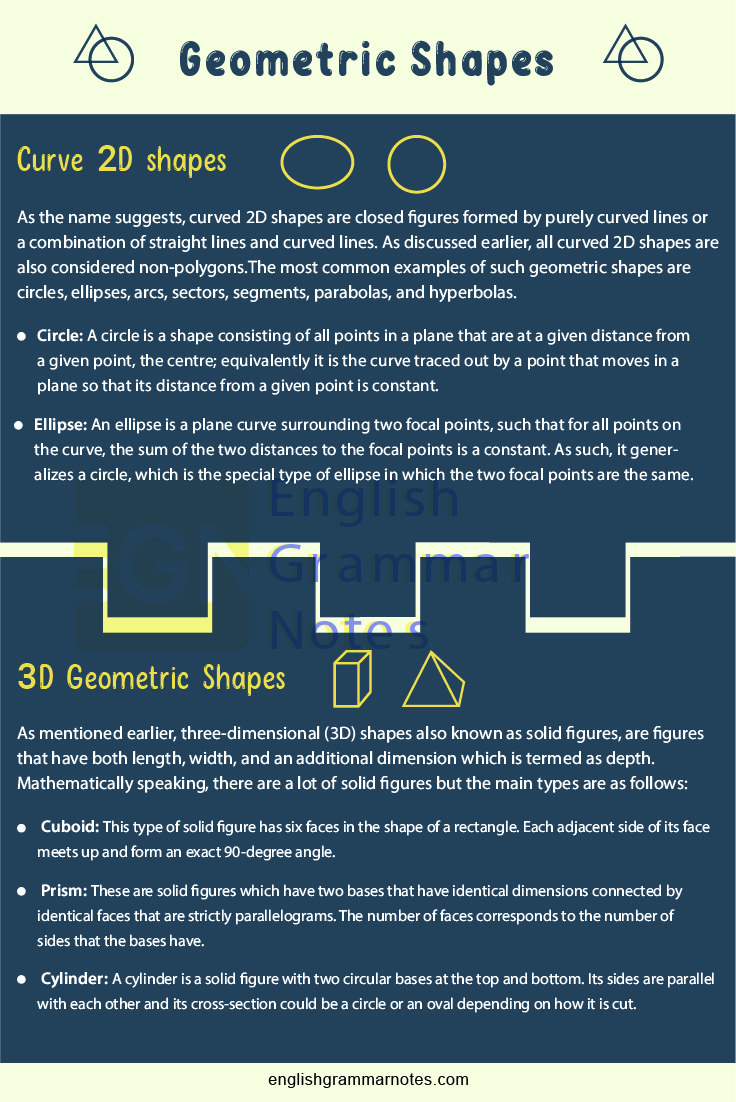# Geometric Shapes | Amazing List of 2D & 3D Shapes in English

Geometric Shapes: By definition, geometric shapes are the purest form of an object or figure in a sense that no matter how much it is moved, rotated, enlarged, or reflected in the mirror, it will just stay in the same form as it was originally when you still did not touch it. To put it in even simpler terms, if you say that something is a circle in geometry, no matter what angle you look at it or how much you fiddle around with it, it will still have the property of a geometric circle.

To make it simpler, we made a list of types of geometric shapes which are divided into two major groups depending on their dimensions. The first group consists a list of two-dimensional (2D) shapes, which have length and width while the second group consists of example of three-dimensional (3D) shapes that have length, width, and depth.

## List of Types of Geometric Shapes

### Description of Types of Geometric Shapes on the list

#### 2D Geometric Shapes

As explained above, 2D geometric shapes have length and width. Under 2D shapes, there are two further classifications namely polygons and non-polygons. Polygons are two-dimensional geometric shapes that are made up of straight lines that meet up at one of their endpoints and forms a closed figure. Non-polygons on the other hand, are closed figures made up of curved lines or a combination of straight lines and curved lines.

#### 1. Triangles

A triangle is a type of polygon that exactly has three sides and three vertices, or corners. There are different kinds of triangles and they are classified through the length of their sides or through their interior angles.

#### A. Equilateral Triangle

It is a kind of triangle with all three sides having the same length. an equilateral triangle is a triangle in which all three sides have the same length. In the familiar Euclidean geometry, an equilateral triangle is also equiangular; that is, all three internal angles are also congruent to each other and are each 60°.

#### B. Isosceles Triangle

It is a kind of triangle that exactly has two sides of equal length. an isosceles triangle is a triangle that has two sides of equal length. Sometimes it is specified as having exactly two sides of equal length, and sometimes as having at least two sides of equal length, the latter version thus including the equilateral triangle as a special case.

#### C. Scalene Triangle

It is a kind of triangle that has no sides of equal length. A scalene triangle has all its sides of different lengths. Equivalently, it has all angles of different measure.

#### A. Right Triangle

A triangle that has a 90-degree interior angle. The side directly opposite of this angle is called the hypotenuse, which is also the longest side of a right triangle. The relation between the sides and other angles of the right triangle is the basis for trigonometry.

#### B. Oblique Triangle

It is simply a triangle that does not have a 90-degree interior angle. Under oblique triangles are two more types namely:

#### B.1. Acute Triangle

These triangles all have three of their interior angles measuring less than 90 degrees.

#### B.2. Obtuse Triangle

These triangles have one interior angle which measures above 90 degrees.

#### C. Degenerate Triangle

It is a triangle that has an interior angle of 180 degrees. However, it technically looks like a line segment if you try to draw it.Quadrilaterals are polygons that have exactly four sides and four corners. Quadrilaterals are divided into two major types namely, simple and complex.

Simple quadrilaterals are quadrilaterals that do not intersect in themselves. This type is further divided into two which are:

A.1. Convex Quadrilaterals – All quadrilaterals belonging to this type does not have an interior angle of more than 180 degrees. The following are its types:

#### A1.1. Trapezium

A quadrilateral that has no sides that are parallel with each other.

#### A1.2. Trapezoid

A quadrilateral that has exactly one pair of parallel sides.

#### A1.3. Isosceles Trapezoid

A trapezoid that has base angles of equal value.

#### A1.4. Parallelogram

A quadrilateral with two pairs of parallel sides.

#### A1.5. Rhombus

A quadrilateral with four equal sides.

#### A1.6. Square

A type of rhombus which has four right angles.

#### A1.7. Rectangle

A type of parallelogram with all interior angles measuring 90 degrees.

#### A1.8. Kite

A quadrilateral with an interior angle greater than 180 degrees. It only has one type which is called dart.

These quadrilaterals intersect in themselves which makes it look like a simple bow-tie in shape.

#### 3. Concave and Convex Polygons

The main difference between concave and convex polygons lies in the measurement of their interior angles. A convex polygon simply has all of its interior angles measuring less than 180 degrees while a concave polygon has one or more interior angles going beyond 180 degrees.

#### 3.1. Convex Polygon

A convex polygon is a simple polygon (not self-intersecting) in which no line segment between two points on the boundary ever goes outside the polygon.

Equivalently, it is a simple polygon whose interior is a convex set. In a convex polygon, all interior angles are less than or equal to 180 degrees, while in a strictly convex polygon all interior angles are strictly less than 180 degrees.

#### 3.2. Concave Polygon

A simple polygon that is not convex is called concave, non-convex or re-entrant. A concave polygon will always have at least one reflex interior angle—that is, an angle with a measure that is between 180 degrees and 360 degrees exclusive.

#### 4. Regular and Irregular Polygons

These two are other classifications of a polygon wherein regular polygons both have sides of equal lengths and interior angles of equal measurement.

The most common examples of a regular polygon include an equilateral triangle, a square, a pentagon, and hexagons, and octagons. Irregular polygons, on the other hand, are figures which do not satisfy both conditions to be considered as a regular polygon.

#### 4.1. Regular Polygons

a regular polygon is a polygon that is equiangular (all angles are equal in measure) and equilateral (all sides have the same length). Regular polygons may be either convex or star.

#### 4.2. Irregular Polygons

Irregular polygons are polygons that have unequal angles and unequal sides, as opposed to regular polygons which are polygons that have equal sides and equal angles.#### 5. Curve 2D shapes

As the name suggests, curved 2D shapes are closed figures formed by purely curved lines or a combination of straight lines and curved lines. As discussed earlier, all curved 2D shapes are also considered non-polygons.

The most common examples of such geometric shapes are circles, ellipses, arcs, sectors, segments, parabolas, and hyperbolas.

#### 5.1. Circle

A circle is a shape consisting of all points in a plane that are at a given distance from a given point, the centre; equivalently it is the curve traced out by a point that moves in a plane so that its distance from a given point is constant.

#### 5.2. Ellipse

an ellipse is a plane curve surrounding two focal points, such that for all points on the curve, the sum of the two distances to the focal points is a constant. As such, it generalizes a circle, which is the special type of ellipse in which the two focal points are the same.

#### 5.3. Sectors

A circular sector, also known as circle sector or disk sector, is the portion of a disk (a closed region bounded by a circle) enclosed by two radii and an arc, where the smaller area is known as the minor sector and the larger being the major sector.

#### 5.4. Parabola

a parabola is a plane curve which is mirror-symmetrical and is approximately U-shaped. It fits several superficially different mathematical descriptions, which can all be proved to define exactly the same curves.

#### 5.5. Hyperbola

A hyperbola has two pieces, called connected components or branches, that are mirror images of each other and resemble two infinite bows. The hyperbola is one of the three kinds of conic sections, formed by the intersection of a plane and a double cone.#### 3D Geometric Shapes

As mentioned earlier, three-dimensional (3D) shapes also known as solid figures, are figures that have both length, width, and an additional dimension which is termed as depth. Mathematically speaking, there are a lot of solid figures but the main types are as follows:

#### 1. Cuboid

This type of solid figure has six faces in the shape of a rectangle. Each adjacent side of its face meets up and form an exact 90-degree angle.

#### 2. Parallelepiped

This figure is just like a cuboid except that its faces are parallelograms instead of rectangles. Hence, its adjacent faces do not create 90-degree angles.

#### 3. Rhombohedron

This figure is a parallelepiped with all of its edges having the same length. It is a special case of a parallelepiped where all edges are the same length. It can be used to define the rhombohedral lattice system, a honeycomb with rhombohedral cells.

#### 4. Polyhedron

These are any solid figures which have polygonal faces that are flat as well as having sharp corners and straight edges.

#### 5. Prism

These are solid figures which have two bases that have identical dimensions connected by identical faces that are strictly parallelograms. The number of faces corresponds to the number of sides that the bases have.

#### 6. Cone

A solid figure with a circular base that smoothly tapers to a point which is called a vertex. A cone is formed by a set of line segments, half-lines, or lines connecting a common point, the apex, to all of the points on a base that is in a plane that does not contain the apex.

#### 7. Cylinder

A cylinder is a solid figure with two circular bases at the top and bottom. Its sides are parallel with each other and its cross-section could be a circle or an oval depending on how it is cut.

#### 8. Ellipsoid

This figure is made by rotating an ellipse on its own axes. An ellipsoid is a quadric surface; that is, a surface that may be defined as the zero set of a polynomial of degree two in three variables.

#### 9. Lemon

This figure is made by rotating a circular arc on its major axis. A lemon is a geometric shape, constructed as the surface of revolution of a circular arc of angle less than half of a full circle, rotated about an axis passing through the endpoints of the lens (or arc).

#### 10. Hyperboloid

This solid figure is created when a hyperbola is rotated in one of its principal axes. A hyperboloid is the surface obtained from a hyperboloid of revolution by deforming it by means of directional scalings, or more generally, of an affine transformation.

#### 11. Platonic Solids

These solids are regular, convex polyhedrons made up of faces that are regular polygons each. In geometry, only five solid figures have met these criteria and they are as follows:

#### 11.1. Tetrahedron- Four faces

A tetrahedron (plural: tetrahedra or tetrahedrons), also known as a triangular pyramid, is a polyhedron composed of four triangular faces, six straight edges, and four vertex corners. The tetrahedron is the simplest of all the ordinary convex polyhedra and the only one that has fewer than 5 faces.

#### 11.2. Cube- Six faces

A cube is a three-dimensional solid object bounded by six square faces, facets or sides, with three meeting at each vertex. The cube is the only regular hexahedron and is one of the five Platonic solids. It has 6 faces, 12 edges, and 8 vertices.

#### 11.3. Octahedron-Eight faces

An octahedron (plural: octahedra, octahedrons) is a polyhedron with eight faces, twelve edges, and six vertices. The term is most commonly used to refer to the regular octahedron, a Platonic solid composed of eight equilateral triangles, four of which meet at each vertex.

#### 11.4. Dodecahedron- Twelve faces

A dodecahedron or duodecahedron is any polyhedron with twelve flat faces. The most familiar dodecahedron is the regular dodecahedron with regular pentagons as faces, which is a Platonic solid.

#### 11.5. Icosahedron- Twenty faces

An icosahedron is a polyhedron with 20 faces. The name comes from Ancient Greek ‘twenty’ and from Ancient Greek ‘ seat’. The plural can be either “icosahedra” or “icosahedrons”. There are infinitely many non-similar shapes of icosahedra, some of them being more symmetrical than others.